# 如何验证会员系统中用户的邮箱是否真实存在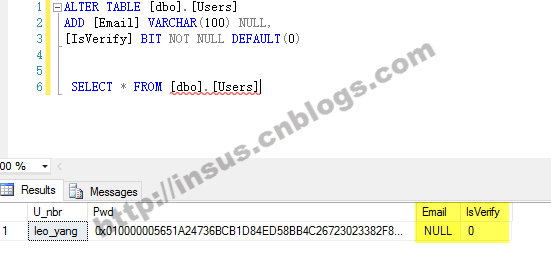```ALTER TABLE [dbo].[Users]
[IsVerify] BIT NOT NULL DEFAULT(0)
SELECT * FROM [dbo].[Users]```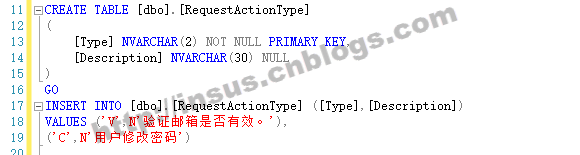```CREATE TABLE [dbo].[RequestActionType]
(
[Type] NVARCHAR(2) NOT NULL PRIMARY KEY,
[Description] NVARCHAR(30) NULL
)
GO
INSERT INTO [dbo].[RequestActionType] ([Type],[Description])
VALUES ('V',N'验证邮箱是否有效。'),
('C',N'用户修改密码')```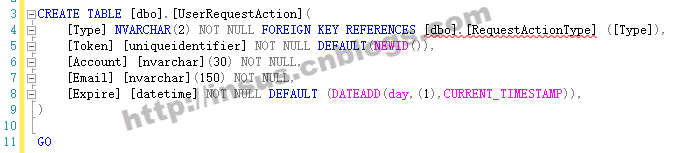```CREATE TABLE [dbo].[UserRequestAction](
[Type] NVARCHAR(2) NOT NULL FOREIGN KEY REFERENCES [dbo].[RequestActionType] ([Type]),
[Token] [uniqueidentifier] NOT NULL DEFAULT(NEWID()),
[Account] [nvarchar](30) NOT NULL,
[Email] [nvarchar](150) NOT NULL,
[Expire] [datetime] NOT NULL DEFAULT (DATEADD(day,(1),CURRENT_TIMESTAMP)),
)
GO
Source Code```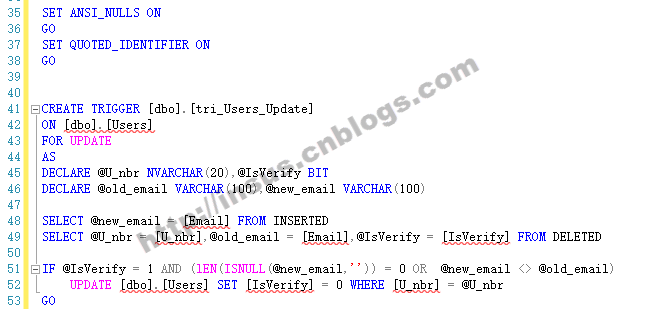```CREATE TRIGGER [dbo].[tri_Users_Update]
ON [dbo].[Users]
FOR UPDATE
AS
DECLARE @U_nbr NVARCHAR(20),@IsVerify BIT
DECLARE @old_email VARCHAR(100),@new_email VARCHAR(100)
SELECT @new_email = [Email] FROM INSERTED
SELECT @U_nbr = [U_nbr],@old_email = [Email],@IsVerify = [IsVerify] FROM DELETED
IF @IsVerify = 1 AND (lEN(ISNULL(@new_email,'')) = 0 OR @new_email <> @old_email)
UPDATE [dbo].[Users] SET [IsVerify] = 0 WHERE [U_nbr] = @U_nbr
GO
Source Code``````CREATE PROCEDURE [dbo].[usp_UserRequestAction_Request]
(
@Type NVARCHAR(2),
@U_nbr NVARCHAR(20)
)
AS
IF NOT EXISTS(SELECT TOP 1 1 FROM [dbo].[Users] WHERE [U_nbr] = @U_nbr)
BEGIN
RAISERROR(N'帐号错误或不存存在，请联系系统管理员。',16,1)
RETURN
END
DECLARE @Email NVARCHAR(100)
SELECT @Email = [Email] FROM [dbo].[Users] WHERE [U_nbr] = @U_nbr
IF EXISTS(SELECT TOP 1 1 FROM [dbo].[UserRequestAction] WHERE [Type] = @Type AND [Account] = @U_nbr AND [Email] = @Email)
UPDATE [dbo].[UserRequestAction] SET [Token] = NEWID(),[Expire] = DATEADD(day,(1),CURRENT_TIMESTAMP) WHERE [Type] = @Type AND [Account] = @U_nbr AND [Email] = @Email
ELSE
INSERT INTO [dbo].[UserRequestAction] ([Type],[Account],[Email]) VALUES (@Type,@U_nbr,@Email)
GO
Source Code```

Ok,还差2个存储过程，第一个是更新IsVerify字段值：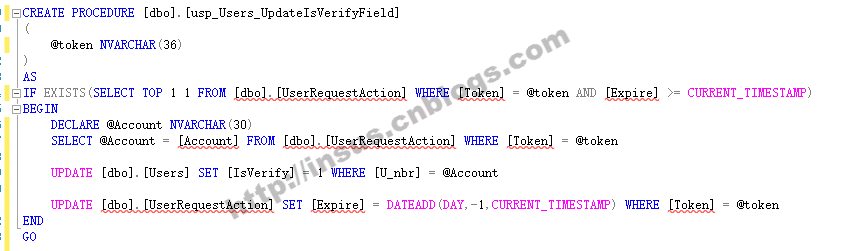```CREATE PROCEDURE [dbo].[usp_Users_UpdateIsVerifyField]
(
@token NVARCHAR(36)
)
AS
IF EXISTS(SELECT TOP 1 1 FROM [dbo].[UserRequestAction] WHERE [Token] = @token AND [Expire] >= CURRENT_TIMESTAMP)
BEGIN
DECLARE @Account NVARCHAR(30)
SELECT @Account = [Account] FROM [dbo].[UserRequestAction] WHERE [Token] = @token
UPDATE [dbo].[Users] SET [IsVerify] = 1 WHERE [U_nbr] = @Account
UPDATE [dbo].[UserRequestAction] SET [Expire] = DATEADD(DAY,-1,CURRENT_TIMESTAMP) WHERE [Token] = @token
END
GO
Source Code```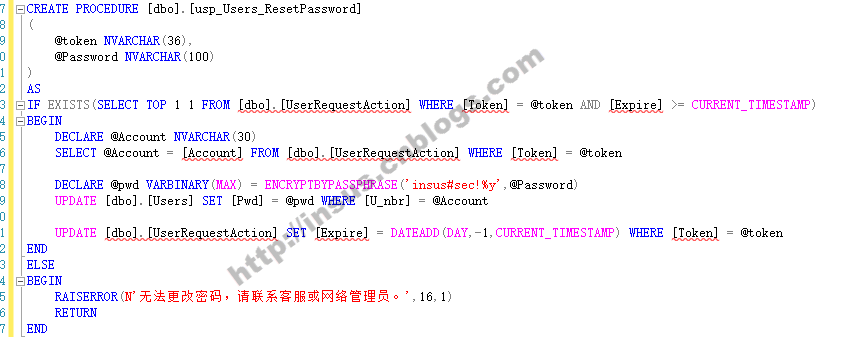```CREATE PROCEDURE [dbo].[usp_Users_ResetPassword]
(
@token NVARCHAR(36),
)
AS
IF EXISTS(SELECT TOP 1 1 FROM [dbo].[UserRequestAction] WHERE [Token] = @token AND [Expire] >= CURRENT_TIMESTAMP)
BEGIN
DECLARE @Account NVARCHAR(30)
SELECT @Account = [Account] FROM [dbo].[UserRequestAction] WHERE [Token] = @token
UPDATE [dbo].[Users] SET [Pwd] = @pwd WHERE [U_nbr] = @Account
UPDATE [dbo].[UserRequestAction] SET [Expire] = DATEADD(DAY,-1,CURRENT_TIMESTAMP) WHERE [Token] = @token
END
ELSE
BEGIN
RAISERROR(N'无法更改密码，请联系客服或网络管理员。',16,1)
RETURN
END
Source Code```

Spark SQL数据加载和保存实例讲解

SqlServer高版本数据备份还原到低版本

SQLServer 2008数据库降级到2005低版本